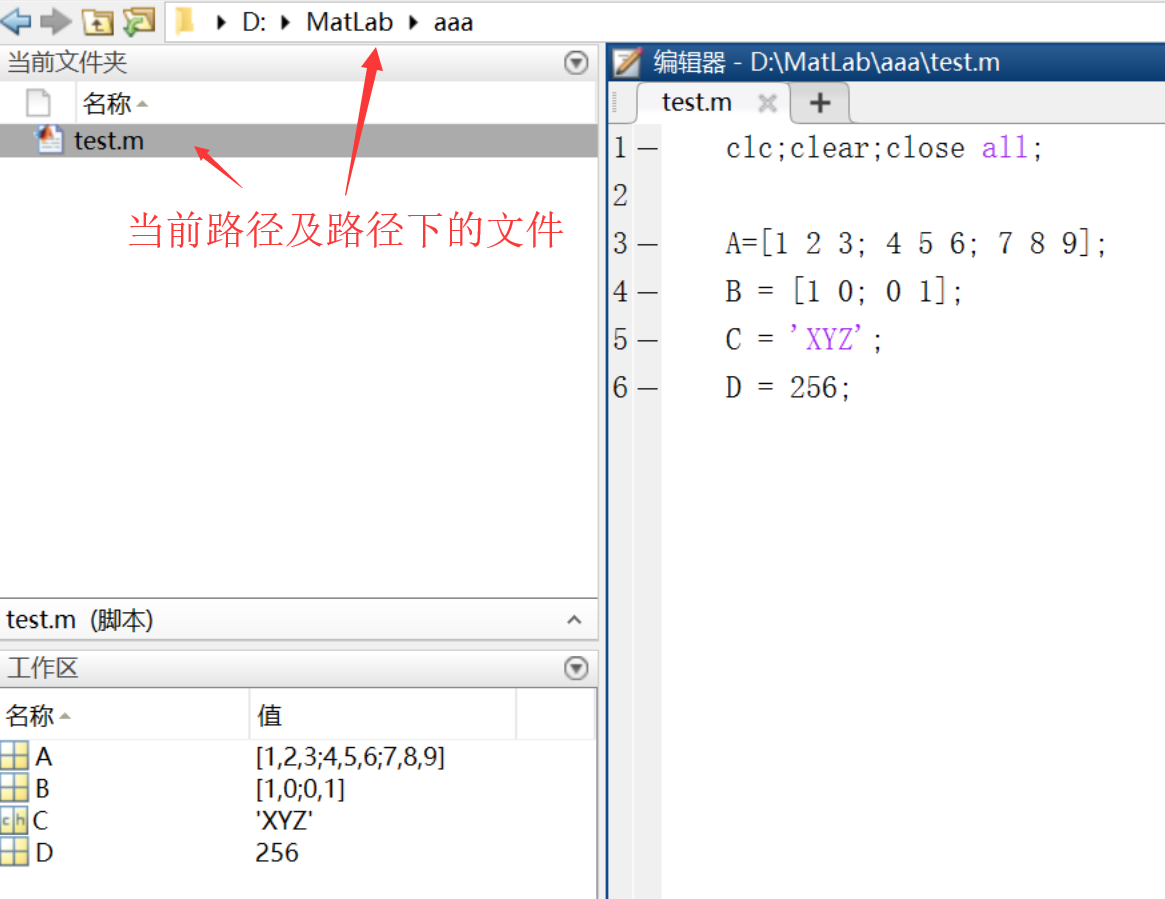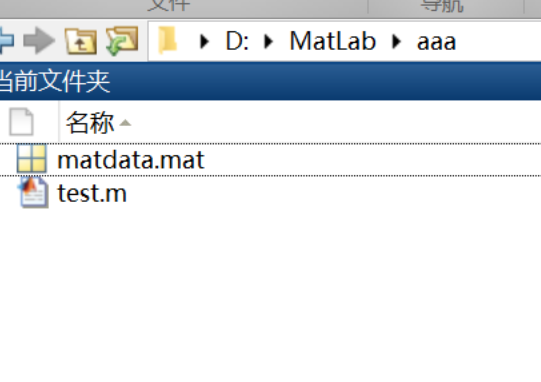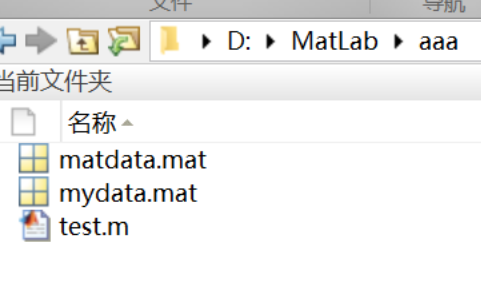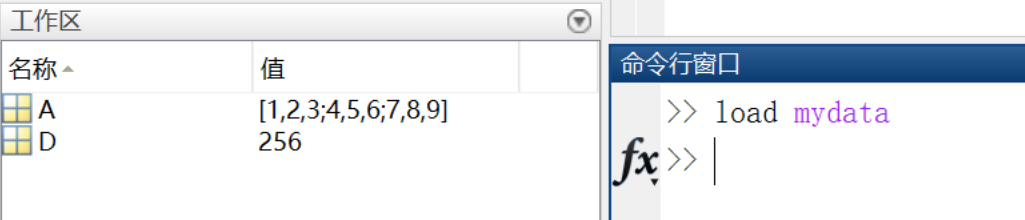• 一、Mat初始化 1.使用Mat构造函数 //方法一： Mat M( 2, 2, CV_8UC3, Scalar(0,255,0) );//其实是2*6的矩阵，因为每个元素有3个通道。 Mat M1( 2, 2, CV_8UC1，Scalar(0) );//单通道 //方法二： int sz = {2, 2, ...
一、Mat初始化 1.使用Mat构造函数
//方法一：
Mat M( 2, 2, CV_8UC3, Scalar(0,255,0) );//其实是2*6的矩阵，因为每个元素有3个通道。
Mat M1( 2, 2, CV_8UC1，Scalar(0) );//单通道
//方法二：
int sz = {2, 2, 2};
Mat L( 3, sz, CV_8UC(1), Scalar::all(0) );

2.为已存在的IplImage指针创建信息头
IplImage* img = cvLoadImage("1.jpg",1);
Mat test(img);

3.利用create函数
M.create( 4, 4, CV_8UC2);//CV_8UC2里面的2表示2通道

4.采用Matlab形式的初始化方式
Mat E = Mat::eye(4, 4, CV_64F);
Mat O = Mat::ones(2, 3, CV_32F);
Mat Z = Mat::zeros(3, 3, CV_8UC1);

5.Mat矩阵比较小时，学会直接赋值的方法，即用Mat_
Mat C =(Mat_<double>(3,3)<<0,-1,0,-1,5,-1,0,-1,0);//直接赋初始值的方法

6.使用数组或指针初始化
Mat (int rows, int cols, int type, void *data, size_t step=AUTO_STEP)
Mat (Size size, int type, void *data, size_t step=AUTO_STEP)
example：
int a = { 1, 2, 3, 4, 5, 6};
Mat m1(2,3,CV_32S,a);   //float 对应的是CV_32F,double对应的是CV_64F
cout << m1 << endl;

参考链接1
二、Mat访问
Mat和Matlab里的数组格式有点像，但一般是二维向量，如果是灰度图，一般存放"uchar"类型；如果是RGB彩色图，存放"Vec3b"类型。首先先看看图像的是怎么存储的。
单通道图像多通道图像单通道灰度图数据存放格式：多通道的图像中，每列并列存放通道数量的子列，如RGB三通道彩色图：opencv中，由于使用Mat.at访问数据时，必须正确填写相应的数据类型，因此必须弄清楚opencv中的数据类型与我们常用数据类型一一对应关系。

Mat_<uchar>---------CV_8U
Mat_<char>-----------CV_8S
Nat_<short>---------CV_16S
Mat_<ushort>--------CV_16U
Mat_<int>-----------CV_32S
Mat_<float>----------CV_32F
Mat_<double>--------CV_64F

CV_8U 8位无符号整数 （0…..255）  CV_8S 8 位符号整数 （-128…..127）  CV_16U 16 位无符号整数 （0……65535）  CV_16S 16 位符号整数 （-32768…..32767）  CV_32S 32 位符号整数 （-2147483648……2147483647）  CV_32F 32 位浮点数 （-FLT_MAX ………FLT_MAX，INF，NAN)  CV_64F 64 位浮点数 （-DBL_MAX ……….DBL_MAX，INF，NAN)
下面主要记录三种常见的访问Mat的方式：1.at访问 2.ptr访问 3.data访问 Mat中不管是以at访问还是ptr访问，都是行优先 ，先Y轴后X轴（即先行后列）
//方法1：
t = (double)getTickCount();
Mat img1(1000, 1000, CV_32F);

for (int i=0; i<1000; i++)
{
for (int j=0; j<1000; j++)
{
img1.at<float>(i,j) = 3.2f;
}
}
t = (double)getTickCount() - t;
printf("in %gms\n", t*1000/getTickFrequency());
//***方法2************************************************************
t = (double)getTickCount();
Mat img2(1000, 1000, CV_32F);

for (int i=0; i<1000; i++)
{
for (int j=0; j<1000; j++)
{
img2.ptr<float>(i)[j] = 3.2f;
}
}
t = (double)getTickCount() - t;
printf("in %gms\n", t*1000/getTickFrequency());
//***方法3************************************************************
t = (double)getTickCount();
Mat img3(1000, 1000, CV_32F);
float* pData = (float*)img3.data;

for (int i=0; i<1000; i++)
{
for (int j=0; j<1000; j++)
{
*(pData) = 3.2f;
pData++;
}
}
t = (double)getTickCount() - t;
printf("in %gms\n", t*1000/getTickFrequency());
//***方法3************************************************************
t = (double)getTickCount();
Mat img4(1000, 1000, CV_32F);

for (int i=0; i<1000; i++)
{
for (int j=0; j<1000; j++)
{
((float*)img3.data)[i*1000+j] = 3.2f;
}
}
t = (double)getTickCount() - t;
printf("in %gms\n", t*1000/getTickFrequency());

对比几种方法，速度测试如下：

测试结果
DebugRelease方法1139.06ms2.51ms方法266.28ms2.50ms方法3-14.95ms2.28ms方法3-25.11ms1.37ms
可以看出在debug版本下测试，不同访问方法差别比较大，Release下还好，而且也可以采用和指针结合的方式来增加效率，比如
/*********加强版********/
t = (double)getTickCount();
Mat img5(1000, 1000, CV_32F);
float *pData1;
for (int i=0; i<1000; i++)
{
pData1=img5.ptr<float>(i);
for (int j=0; j<1000; j++)
{
pData1[j] = 3.2f;
}
}
t = (double)getTickCount() - t;
printf("in %gms\n", t*1000/getTickFrequency());


DebugRelease加强版5.74ms2.43ms

参考链接2 参考链接3 参考链接4 参考链接5 参考链接6
多通道Mat访问 用at进行多通道访问，对应的数据类型如下： typedef Vec<uchar, 2> Vec2b; //无符号双通道 CV_8U:0~255 typedef Vec<uchar, 3> Vec3b; //无符号3通道 typedef Vec<uchar, 4> Vec4b; typedef Vec<short, 2> Vec2s; //short型双通道 CV_16S:-32768~32767 typedef Vec<short, 3> Vec3s; typedef Vec<short, 4> Vec4s; typedef Vec<int, 2> Vec2i; //整型双通道 CV_32S:-2147483648~2147483647 typedef Vec<int, 3> Vec3i; typedef Vec<int, 4> Vec4i; typedef Vec<float, 2> Vec2f; //浮点型双通道 CV_32F:1.1810-38~3.401038 typedef Vec<float, 3> Vec3f; typedef Vec<float, 4> Vec4f; typedef Vec<float, 6> Vec6f; typedef Vec<double, 2> Vec2d; //double型双通道 CV_64F:2.2310-308~1.7910308 typedef Vec<double, 3> Vec3d; typedef Vec<double, 4> Vec4d; typedef Vec<double, 6> Vec6d;
示例程序，创建及访问双通道Mat
#include <opencv2/core/core.hpp>
#include <iostream>

using namespace std;
using namespace cv;

int main()
{
int i, j;
Mat dimg(3, 5, CV_16SC2,Scalar(-5,5));//构造一个3*5的short型双通道矩阵
cout << "原矩阵" << endl << dimg << endl;
short int a=1,b=15;
for (i = 0; i < dimg.rows; i++)
{
for (j = 0; j < dimg.cols; j++)
{
dimg.at<Vec2s>(i, j) = a;//at<type>其中type由矩阵的类型确定
dimg.at<Vec2s>(i, j) = b;
}
}

cout << "改变后矩阵" << endl << dimg << endl;
system("pause");
}


参考链接6 参考链接7
欢迎大家点赞、收藏、评论，让更多的人看到哦~
展开全文• ## Matlab读写.mat数据

万次阅读 多人点赞 2018-06-08 08:57:44
.mat文件的方便之处在于，它可以连同数据的变量名一同保存下来（这一点在程序调试的过程中很方便），并且不需要控制数据的存储格式，matlab会自动保存并区分我们所存储的内容。利用load和save指令可以实现对.mat文件的读写。

（1）利用save指令写.mat文件
首先在Editor内建立一些示例变量，如下所示：
A = [1 2 3; 4 5 6; 7 8 9];  B = [1 0; 0 1];  C = 'XYZ';  D = 256;
此时运行matlab，可以在Workspace内看到这些变量，如下图所示：此时添加下述程序：（可以在Command Window内写，也可以在Editor内写）

save matdata;
则名称为matdata.mat的文件保存在当前默认的路径下了，如下图所示：此时Workspace内所有的数据连同他们的变量名称都保存在matdata.mat文件内，
如果只想保存指定的数据，则可以使用如下命令：
save mydata A D;
此时被指定的数据A和D被保存在mydata.mat文件内，保存路径也是当前默认的，如下图所示：若要保存全部数据中的某一部分，则可以调用如下命令：

save ('filename', 'dataname');
其中filename是.mat的名称；dataname是需要保存的数据的名字。

利用上面的指令就读入了mydata.mat内的数据，可以看到此时的Workspace内只有A和D的数据，如下图所示：展开全文• ## OpenCV实现Mat与vector，Mat与数组互转

万次阅读 多人点赞 2018-05-09 17:09:38
OpenCV实现Mat与vector互转 【尊重原创，转载请注明出处】https://blog.csdn.net/guyuealian/article/details/80253066 1、Mat与vector互转 下面是鄙人实现的Mat与vector互转的方法，需要注意的是vector转Mat时...
OpenCV实现Mat与vector互转
【尊重原创，转载请注明出处】https://blog.csdn.net/guyuealian/article/details/80253066
1、Mat与vector互转
下面是鄙人实现的Mat与vector互转的方法，需要注意的是vector转Mat时，使用reshape()后，必须clone()一份，否则返回的结果出错，关于这方面的原因，可以参考鄙人的博客：https://blog.csdn.net/guyuealian/article/details/80252853
#include <opencv2/opencv.hpp>

using namespace cv;
using namespace std;

/***************** Mat转vector **********************/
template<typename _Tp>
vector<_Tp> convertMat2Vector(const Mat &mat)
{
return (vector<_Tp>)(mat.reshape(1, 1));//通道数不变，按行转为一行
}

/****************** vector转Mat *********************/
template<typename _Tp>
cv::Mat convertVector2Mat(vector<_Tp> v, int channels, int rows)
{
cv::Mat mat = cv::Mat(v);//将vector变成单列的mat
cv::Mat dest = mat.reshape(channels, rows).clone();//PS：必须clone()一份，否则返回出错
return dest;
}

int main()
{
/* char ->CV_8SC
* unsigned char,uchar ->CV_8UC
* unsigned short int,ushort->CV_16UC
* short int->CV_16SC
* int   ->CV_32SC
* float ->CV_32FC
* double->CV_64FC
*/
uchar arr = { { 1, 1,1 },{ 2, 2,2 },{ 3, 3,3 },{ 4,4, 4 } };
cv::Mat srcData(4, 3, CV_8UC1, arr);
cout << "srcData=\n" << srcData << endl;

vector<uchar> v = convertMat2Vector<uchar>(srcData);
cv::Mat dest = convertVector2Mat<uchar>(v, 1, 4);//把数据转为1通道，4行的Mat数据
cout << "dest=\n" << dest << endl;

system("pause");
waitKey();
return 0;
}

运行结果：
srcData=
[  1,   1,   1;
2,   2,   2;
3,   3,   3;
4,   4,   4]
dest=
[  1,   1,   1;
2,   2,   2;
3,   3,   3;
4,   4,   4]
2、Mat与数组互转
利用Mat来存储数据，避免使用数组等操作
cv::Mat mean = (cv::Mat_<float>(2, 1) << 0.4404, 0.3111);
cout << "mean=" << mean << endl;
float a=mean.at<float>(0, 0);
float b = mean.at<float>(0, 0);
将数组内容传递给Mat，示例代码：
unsigned char cbuf[height][width];
cv::Mat img(height, width, CV_8UC1, (unsigned char*)cbuf);
将Mat中的内容传递给数组，如果Mat中的数据是连续的，那么对于传递到一维vector我们可以这样：
std::vector<uchar> array(mat.rows*mat.cols);
if (mat.isContinuous())
array = mat.data;
同样的，传递到一维数组我们可以这样
unsigned char *array=new unsigned char[mat.rows*mat.cols];

if (mat.isContinuous())
array = mat.data;
对于二维vector的传值，我们可以这样处理​​​​​​​
uchar **array = new uchar*[mat.rows];
for (int i=0; i<mat.rows; ++i)
array[i] = new uchar[mat.cols];

for (int i=0; i<mat.rows; ++i)
array[i] = mat.ptr<uchar>(i);

RGB图与数组转换（3维矩阵）
    BYTE* iPtr = new BYTE [height*width*3];
for(int i=0;i<height;i++)
{
for(int j=0;j<width;j++)
{
for(int k=0;k<3;k++)
{
iPtr[i*width*3+j*3+k] = img.at<Vec3b>(i,j)[k];
}
}
}
其中，img是一个3维uchar的Mat，Vec3b代表3个uchar，对于灰度图、4维矩阵等，只要把通道数和at的数据类型改一下就可以套用以上格式。还有一点千万注意，Mat的（i，j）是按（行，列）的规则，而图像中则是（高，宽），跟Size(x,y)，Rect(x,y)的（x，y）是不同的
4字节对齐的情况
http://lib.csdn.net/article/opencv/24030
int nr=image.rows;
int nc=image.cols;
if(image.isContinuous())
{
nr=1;
nc=nc*image.rows*image.channels();
}
for(int i=0;i<nr;i++)
{
const uchar* inData=image.ptr<uchar>(i);
uchar* outData=outImage.ptr<uchar>(i);
for(int j=0;j<nc;j++)
{
*outData++=*inData++;
}
}  
例如保存BMP格式的图像时，BMP要求图像数据按四字节对齐，此时就需要对Mat中的数据进行补零 对齐方法就是在每一行尾部补零，零的个数可能是1~3个
但其实大部分时候，Mat的内存都是连续的，只有极个别时候需要担心这个问题，这里有说明，和这里
展开全文• ## Mat &与Mat区别

千次阅读 2020-01-17 20:40:45
Mat homo_filter(Mat src, Mat homo_filter_img); 调用后无法继续操作 Mat homo_filter(Mat src, Mat& homo_filter_img); 加上&后才可以imshow imwrite int main() { Mat src = cv::imread("1.jpg"); ...
Mat homo_filter(Mat src, Mat  homo_filter_img);

调用后无法继续操作
Mat homo_filter(Mat src, Mat& homo_filter_img);

加上&后才可以imshow imwrite
int main() {
imshow("src", src);
Mat homo_filter_img;
homo_filter(src,homo_filter_img);

imshow("result", homo_filter_img);
imwrite("result.jpg",homo_filter_img);
waitKey(0);
return 0;
}

void mysort(mat a)的a是值传递方式 值传递： 形参是实参的拷贝，改变形参的值并不会影响外部实参的值。从被调用函数的角度来说，值传递是单向的（实参->形参），**参数的值只能传入， 不能传出。**当函数内部需要修改参数，并且不希望这个改变影响调用者时，采用值传递。 void mysort(mat& a)的&a是引用传递方式 引用传递： 形参相当于是实参的“别名”，对形参的操作其实就是对实参的操作，在引用传递过程中，被调函数的形式参数虽然也作为局部变量在栈中开辟了内存空间，但是这时存放的是由主调函数放进来的实参变量的地址。被调函数对形参的任何操作都被处理成间接寻址，即通过栈中存放的地址访问主调函数中的实参变量。正因为如此，被调函数对形参做的任何操作都影响了主调函数中的实参变量。
展开全文• mac mat工具 点击查看包内容--》mat.app/Contents/MacOS /MemoryAnalyzer 双击MemoryAnalyzer打开Python
• Unable to read MAT-file …/.matlab/R2017b/matlabprefs.mat: not a binary MAT-file. Try LOAD -ASCII to read as text. 解决方法 按照他提示的MAT-file 路径下，将matlabprefs.mat删除即可。 ...matlab 关闭
• 关键字：CV_DbgAssert(CV_ELEM_SIZE1(traits::Depth<...关于MatMat_ 使用相机标定得到的外参数矩阵时出了点问题，记录一下 问题与解决方案 矩阵cMatrix，类型是Mat_<float> 直接cout<...opencv
• gl-mat3 的的fork的一部分，被分割成较小的部分：该程序包包含glMatrix.mat3 。 用法 mat3 = require('gl-mat3') 将加载模块的所有功能，并将其公开在单个对象上。 请注意，也可能直接从其文件中要求使用任何方法...JavaScript
• ## OpenCV Mat类详解和用法

万次阅读 多人点赞 2017-04-13 16:58:49
OpenCV Mat类详解和用法  我们有多种方法可以获得从现实世界的数字图像：数码相机、扫描仪、计算机体层摄影或磁共振成像就是其中的几种。在每种情况下我们（人类）看到了什么是图像。但是，转换图像到我们的数字...OpenCV
• ## Python解析mat文件

千次阅读 2020-04-10 16:26:08
mat文件 mat文件是matlab的数据存储的标准格式。mat文件是标准的二进制文件,还可以ASCII码形式保存和加载，在MATLAB中打开显示类似于单行EXCEL表格。 很多数据集的标签都是通过mat文件来存储的，为了解析出图片所...
• 提供解决方案-vector初始化后存放Mat，出现Mat矩阵数据同变问题解决方案 vector
• ## Opencv中的Mat与Mat&

千次阅读 2020-04-01 21:25:16
Opencv中的MatMat& Mat img1；//创建一个图像 Mat& img2=img1；//创建img1的引用，修改img2等同于修改img1，二者内存地址相同。 例如： SetMouseCallback(WINDOW_NAME,on_MouseHandle,(void*)&...opencv 计算机视觉
• 一、 hprof 文件转换、 二、 下载 Memory Analyzer ( MAT ) 工具、 三、 Memory Analyzer ( MAT ) 工具打开内存快照、hprof-conv
• 1、Mat--&gt;QImage static QImage cvMat_to_QImage(const cv::Mat &amp;mat ) { switch ( mat.type() ) { // 8-bit, 4 channel case CV_8UC4: { QImage image( mat.data, mat.cols, ma...学习
• C#语言下，官方好像没有提供直接的方式把Bitmap转为Mat,但是可以使用我的这个方式转变格式
• #include <opencv2/highgui/highgui.hpp> #include <opencv2/imgproc/imgproc.hpp> #include <iostream>... Mat r = Mat(10, 4, CV_8UC2); randu(r, Scalar::all(0), Scalar::all(255)).opencv
• ## mac 安装mat

千次阅读 2020-04-07 20:13:43
之前用elipse的时候，mat分析dump文件很方便，换了Mac 也换了idea之后，想使用mat，发现还是有点坑。 一下载 ...mac dump 内存分析
• ## ncnn::Mat转cv::Mat

千次阅读 2020-01-16 15:26:15
ncnn中的数据是float类型, cv::Mat的类型是CV_8UC3, mat.data指定的类型是char *型, 故可以用下标[]直接索引. ncnn中数据的排列格式为(channel, h, w), cv::Mat中数据的排列格式为(h, w, channel). cv::Mat中颜色...
• python读取matlab数据(.mat文件) 我们都知道，matlab是一个非常好用的矩阵计算分析软件，然额，matlab自带的绘图效果极为锯齿，所以，这里分享一个在python中读取matlab处理后的数据.mat文件。 1.首先，我们这里先...matlab python
• ## MAT 下载

千次阅读 2018-02-10 14:24:19
• 合并mat文件 二.拼接矩阵或数据 一.合并mat文件 将如下四个mat文件合并，每个文件为1500行101列，合并完为7500行101列 clc clear all close all %% a=[]; List =dir('C:\Users\Administrator\Desktop\code\...
• ## Mat::ones() 和 Mat::zeros()

万次阅读 多人点赞 2019-01-03 18:50:43
Mat::ones() Mat m = Mat::ones(2, 2, CV_8UC3); 相当于：Mat m = Mat(2, 2, CV_8UC3, 1); // OpenCV replaces 1 with Scalar(1,0,0)相当于每个像素的第一个通道为1，其余两个通道为0； Mat::zeros() Mat m = Mat::...opencv
• ## mat文件

千次阅读 2011-01-28 10:28:00matlab file 存储
• ## MAT分析工具

万次阅读 2019-04-07 15:21:39JAVA JVM 内存分析 Dump 内存泄漏matlab
• 基于MATLAB的代码，将csv格式数据转mat格式数据； 基于MATLAB的代码，将csv格式数据转mat格式数据
• ## MacOS 修改MAT内存

千次阅读 2020-07-12 17:02:53
路径: /Applications/mat.app/Contents/Eclipse/MemoryAnalyzer.ini 参数: -Xms2048m -Xmx2048m -startup ../Eclipse/plugins/org.eclipse.equinox.launcher_1.5.0.v20180512-1130.jar --launcher.library ../...JVM
• Mat origin_Img = Mat::zeros(m_nGoldenHeight, m_nGoldenWidth, CV_8UC1);;uchar* pimage = origin_Img.data;origin_Img.data = pimage;...